## How To Convert Text to Upper Cases(Using VBA) in Excel

This post will show you how to switch from lower case to upper case in Excel. and I am going to show you two different ways of converting text to upper cases using formula or VBA macro in Excel 2013,Excel 2016,Excel 2019 or Excel 365.

If you are familiar using Microsoft Word and you can easily change the text to Upper, Lower, and Title cases using “Change Case” command. But this button is not available in Microsoft Excel. but you can still easily accomplish this in Microsoft Excel as well.

Assume that you have a data set where you want to change all text to Upper cases, you can do it through two methods below.

## Convert Text to Upper Cases using Excel Formula or Function

I have a list of few peoples with their names(first name and last name), and I want to change the case of First Name fields to all upper case. To do this, you can use Upper function of Microsoft Excel. just do the following steps:

Step1: you need to insert a blank column between the both First Name and Last Name columns. Just click column B and right click it, and then click on insert from the drop-down menu list. Then a newly column should be created next to Column A.

Step2: Enter the below UPPER formula in Cell B2 and press Enter key.

``=UPPER(A2) ``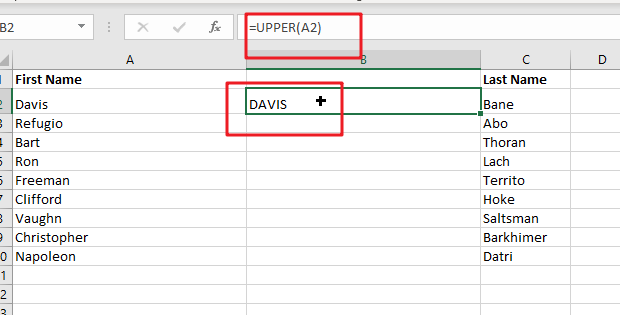Step3: then you need to copy the formula into the remaining cells. or just drag the AutoFill handler in Cell B2 down to other cells to apply this formula.

You can see that there are 2 columns with the same values in your data sets and at this time you can hide the original one.

Note: you can not delete the values from column A, because Column B results depends on Column A. You’d better to hide the actual column or column A.

## Convert Text to Upper Cases using VBA Code

If you want to quickly convert or change text string to uppercase every in your selected range in Excel, and you can use the following short VBA code to achieve the result. Just do the following steps:

Step 1: On current visible worksheet, right click on sheet name tab to load Sheet management menu. Select View CodeMicrosoft Visual Basic for Applications window pops up.

Or you can enter Microsoft Visual Basic for Applications window via Developer->Visual Basic. You can also press Alt + F11 keys simultaneously to open it.

Step 2: In Microsoft Visual Basic for Applications window, enter below code:

```Sub changeUpperCases()
Dim myRange As Range
Dim myworkRange As Range
On Error Resume Next
myTitle = "Convert Text To Upper Cases"
Set myworkRange = Application.Selection
Set myworkRange = Application.InputBox("Select one range that you want to convert to Upper case ", myTitle, myworkRange.Address, Type:=8)
For Each myRange In myworkRange
myRange.Value = VBA.UCase(myRange.Value)
Next
End Sub```

Step 3: Save code, quit Microsoft Visual Basic for Applications.

Step 4: Click Developer->Macros to run Macro.

Step 5: Select the Macro Name ‘changeUpperCases’from the Macro window and click Run.

Step 6: you need to select one range that you wish to convert text string to uppercase, such as: \$A\$1:\$B\$10, then clicking Ok button.

Step 7: Once you have clicked “Ok” button in the step 5, it will go through each row and convert text string to uppercase in your selected range. see the below result:

Note: when you have a VBA Macro in your workbook, and you need to save it as a Macro-enabled file with the .XLSM extension in Excel. Since there are any changes made by the VBA Macro are irreversible, and you’d better to make a backup copy of your workbook or worksheet firstly and then run the VBA code.

You can also copy the contents from Microsoft Excel and then paste them into Microsoft Word. And using the desire Change Case style. And then copy the contents from Microsoft Word and then replace them into your current worksheet.

### Related Functions

• Excel UPPER function
The Excel UPPER function converts all characters in text string to uppercase.The UPPER function is a build-in function in Microsoft Excel and it is categorized as a Text Function.The syntax of the UPPER function is as below:= UPPER (text)…

## How to Convert Uppercase to Lowercase Except the First Letter in Excel

In excel, words can be entered in uppercase, lowercase or mixed. If we want to convert all uppercase to lowercase (except the first letter in some situations), we can use formula with some letter convert related functions to convert them to proper case. This article will introduce you some methods to convert letters from uppercase to lowercase, it can help you to solve your problem.

Precondition:

Prepare below table. To convert uppercase to lowercase for worlds or sentence, actually there are two forms after converting. We can convert uppercase to lowercase but keep the first uppercase for each word; in another way, we can convert uppercase to lowercase but only keep the first uppercase for the first word in the sentence.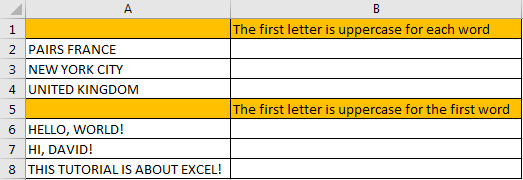## Part 1: Convert Uppercase to Lowercase Except the First Letter for Each Word

Step 1: In B2 enter the formula =PROPER(A2).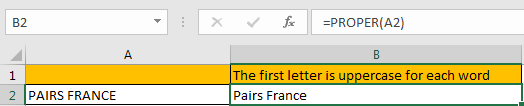For PROPER function, it converts a text or string to proper case, actually it converts the first letter in each word to uppercase, but keeps other letters in lowercase. So, no matter letters are entered in uppercase or lowercase or mixed, it will be finally displayed with the first letter in uppercase with the other letters in lowercase after applying this function.

Step 2: Drag the fill handle down to fill other cells. Verify that uppercase is converted to lowercase properly.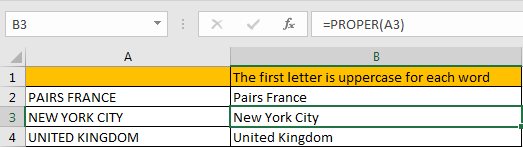## Part 2: Convert Uppercase to Lowercase Except the First Letter for the First Word

Step 1: In B6 enter the formula =UPPER(LEFT(A6,1))&LOWER(RIGHT(A6,LEN(A6)-1)).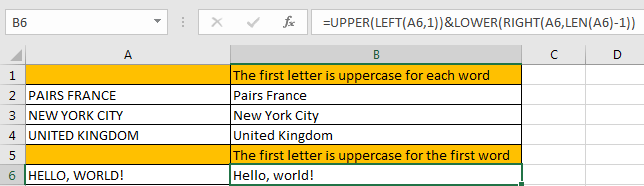This formula is combined with UPPER and LOWER two functions. It is easy to understand. For UPPER function, keep the first letter from left displaying in uppercase; for LOWER function, keep other letters except the first letter from left displaying in lowercase.

Step 2: Drag the fill handle down to fill other cells. Verify that uppercase is converted to lowercase properly.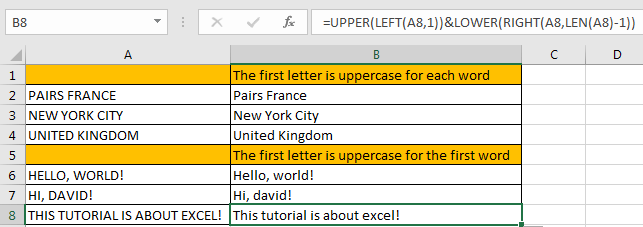### Related Functions

• Excel UPPER function
The Excel UPPER function converts all characters in text string to uppercase.The UPPER function is a build-in function in Microsoft Excel and it is categorized as a Text Function.The syntax of the UPPER function is as below:= UPPER (text)…
• Excel LOWER function
The Excel LOWER function converts all characters in a text string to lowercase.The syntax of the LOWER function is as below:= LOWER  (text)…
• Excel LEFT function
The Excel LEFT function returns a substring (a specified number of the characters) from a text string, starting from the leftmost character.The LEFT function is a build-in function in Microsoft Excel and it is categorized as a Text Function.The syntax of the LEFT function is as below:= LEFT(text,[num_chars])…
• Excel RIGHT function
The Excel RIGHT function returns a substring (a specified number of the characters) from a text string, starting from the rightmost character.The syntax of the RIGHT function is as below:= RIGHT (text,[num_chars])…
• Excel LEN function
The Excel LEN function returns the length of a text string (the number of characters in a text string).The syntax of the LEN function is as below:= LEN(text)…

## How To Force Text String to Upper,Lower, or Proper Case in Excel

This post will guide you how to force test strings to uppercase or lowercase or Proper cases through an Excel formula or data validation in your Excel 2013/2016. How to convert text to upper, lower, or proper case in Excel.

Assuming that you want to force text strings to Upper case when you type words into one cell, and how to do it. You can use an Excel function in Data Validation feature to achieve it. Just do the following steps:

Step1: go to DATA tab, click Data Validation command under the Data Tools group. Then clicking on the Data Validation menu from the drop down menu list. Then the Data Validation window will appear.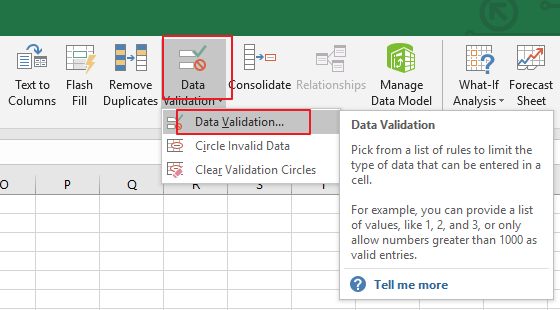Step2: in the Data Validation dialog box, and switch to Settings tab, and click the Allow drop down list and choose Custom value from the drop down menu list.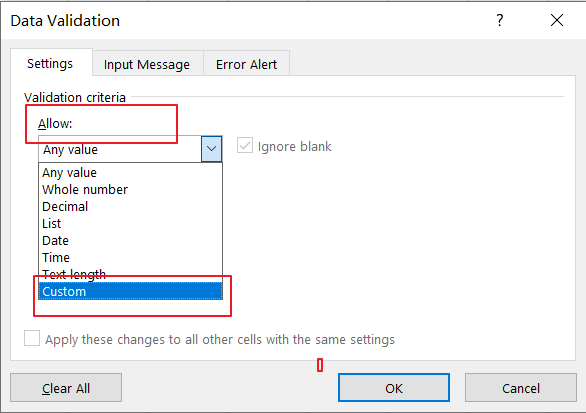Step3: Then type the following formula in the Formula text box in the Data Validation dialog box.

Note:

If you want to convert text string to Upper Case, type the following formula:

=EXACT(UPPER(B1),B1)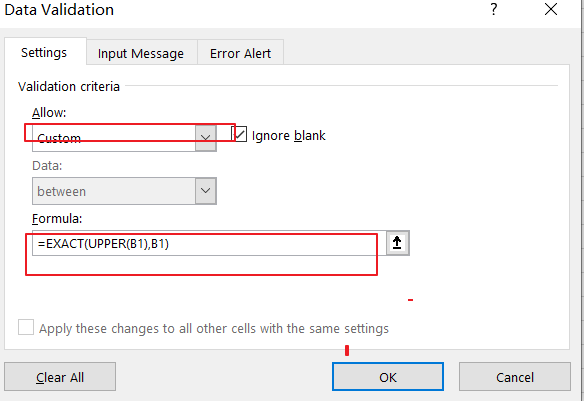If you want to convert text string to Lower Case, just use the following formula:

=EXACT(LOWER(B1),B1)

If you want to convert text string to Proper Case, just use the following formula:

=EXACT(PROPER(B1),B1)

Step4: then you need to switch to Error Alert tab in the Data Validation dialog box. And select Stop value from the Style drop down list box, and type some error message under Error message text box. Then click on Ok button.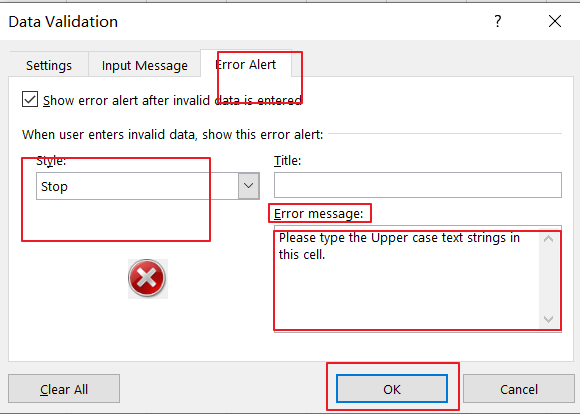Step5: you can try to enter one text string in cell B1 to verify if it take effect.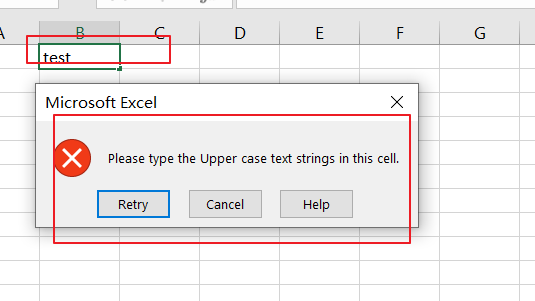You can also use an Excel VBA Macro to achieve the same result of forcing Text string to Upper, Lower or Proper cases in you worksheet. Just do the following steps:

Step1: open your excel workbook and then click on “`Visual Basic`” command under `DEVELOPER` Tab, or just press “`ALT+F11`” shortcut.Step2: then the “`Visual Basic Editor`” window will appear.

Step3: choose on your current worksheet from the left Project Explorer, and double click on it to open the code window, and the copy and paste the following VBA code into it.

For Upper Case:

```Private Sub Worksheet_Change(ByVal Target As Range)

Target.Value = VBA.UCase(Target.Value)

End Sub```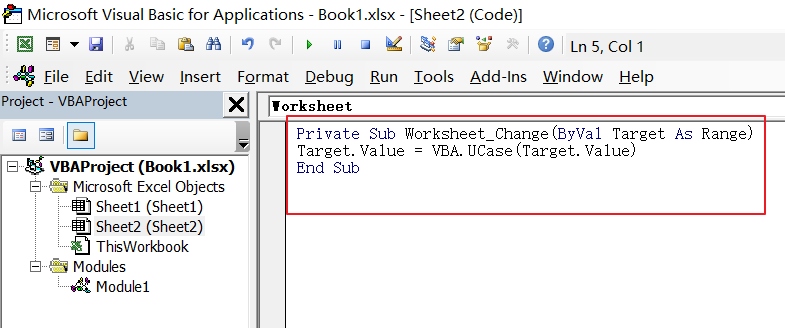For Lower Case:

```Private Sub Worksheet_Change(ByVal Target As Range)

Target.Value = VBA. LCase (Target.Value)

End Sub```

For Proper Case:

```Private Sub Worksheet_Change(ByVal Target As Range)

Target.Value = Application.WorksheetFunction.Proper(Target.Value)

End Sub```

Step4: save and close the current VBA window. And then you can try to enter one text string to verify it. when you type one word when it is lower or proper cases, and it will convert it to Uppercase.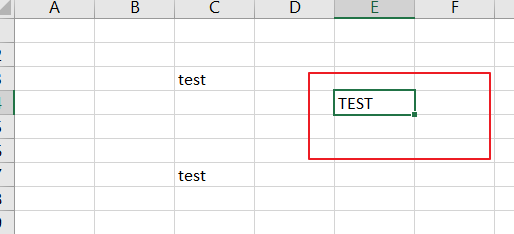### Related Functions

• Excel UPPER function
The Excel UPPER function converts all characters in text string to uppercase.The UPPER function is a build-in function in Microsoft Excel and it is categorized as a Text Function.The syntax of the UPPER function is as below:= UPPER (text)…
• Excel LOWER function
The Excel LOWER function converts all characters in a text string to lowercase.The syntax of the LOWER function is as below:= LOWER  (text)…
• Excel Proper Function
The Excel PROPER function capitalizes the first character in each word of a text string and set other characters to lowercase. The syntax of the PROPER function is as below:= PROPER  (text)…
• Excel EXACT function
The Excel EXACT function compares if two text strings are the same and returns TRUE if they are the same, Or, it will return FALSE.The syntax of the EXACT function is as below:= EXACT (text1,text2)…

## How to Check If a Cell is Uppercase, Lowercases or Capitalize in Excel

This post will guide you how to check if a cell is uppercase in Excel 2013/2016. How do I identify all letters in cells are lowercases or capitalize in Excel. How to change letters to uppercase, lowercases or capitalize first letter for all words in cells in Excel.

## Check If All Letters are Uppercase

If you want to check if all letters in cells are uppercase, you can use a formula based on the EXACT function and the UPPER function. Like this:

`=EXACT(B1,UPPER(B1))`

Type this formula into a blank cell and press Enter key to apply this formula. If All letters are uppercases, and it returns TRUE. Otherwise, it returns FALSE. Then drag the AutoFill Handle down to other cells to apply this formula.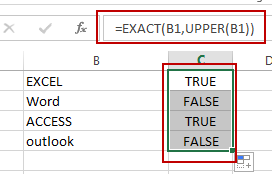Let’ see how this formula works:

The UPPER function will convert all letters to uppercase. And then pass the result to EXACT function as its second argument. And the EXACT function will compare if two values are equal. If equal, returns TRUE. Otherwise, returns FALSE.

## Check If All Letters are Lowercase

If you need to identify all characters in cells are lowercase, you can use a formula based on the LOWER function and the EXACT function. Like this:

`=EXACT(B1,LOWER(B1))`

Type this formula into cell C1, and press Enter key to apply this formula. If all letters are lowercases, and it returns TRUE. Otherwise, it returns FALSE. Then drag the AutoFill Handle down to other cells to apply this formula.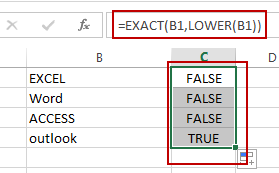The LOWER function converts all letters to lowercase.

## Check If the First Letters are capitalized

If you want to check if the first character in each word is capitalized, you can use a formula based on the EXACT function and the PROPER function. Like this:

`=EXACT(B1,PROPER(B1))`

Type this formula into cell C1, and press Enter key to apply this formula. If all the first letters are capitalized, and it returns TRUE. Otherwise, it returns FALSE. Then drag the AutoFill Handle down to other cells to apply this formula.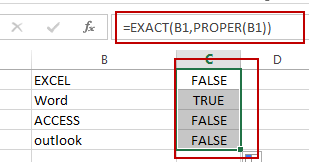The PROPER function will capitalize the first letter in each word in Cell.

### Related Functions

• Excel UPPER function
The Excel UPPER function converts all characters in text string to uppercase.The UPPER function is a build-in function in Microsoft Excel and it is categorized as a Text Function.The syntax of the UPPER function is as below:= UPPER (text)…
• Excel LOWER function
The Excel LOWER function converts all characters in a text string to lowercase.The syntax of the LOWER function is as below:= LOWER  (text)…
• Excel Proper Function
The Excel PROPER function capitalizes the first character in each word of a text string and set other characters to lowercase. The syntax of the PROPER function is as below:= PROPER  (text)…
• Excel EXACT function
The Excel EXACT function compares if two text strings are the same and returns TRUE if they are the same, Or, it will return FALSE.The syntax of the EXACT function is as below:= EXACT (text1,text2)…

## Convert Scientific Notation to Text or a Number in Excel

This post will guide you how to convert scientific notation to text or a number in Excel. How do I convert excel scientific to text with a formula in Excel.

## Convert Scientific Notation to Text or a Number in Excel

Assuming that you have a list of data in range B1:B4 that contain scientific notation numbers and you want to convert those scientific number to text or an integer number. How to achieve it.

You can use the Format cell feature to achieve the result. Just do the following steps:

#1 select the range of cells that you want to convert.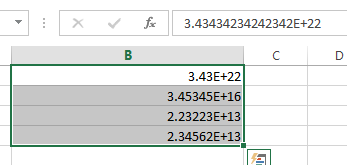#2 right click on it, and select Format Cells from the pop-up menu list. And the Format Cells dialog will open.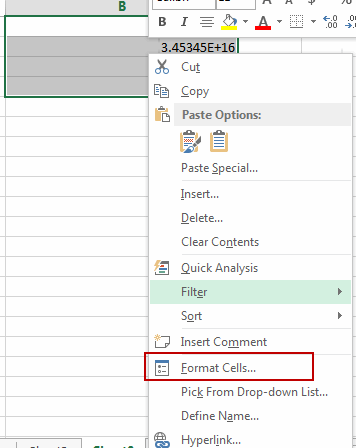#3 switch to Number tab, click Custom under Category list box. And type 0 in the type text box. Then click OK button.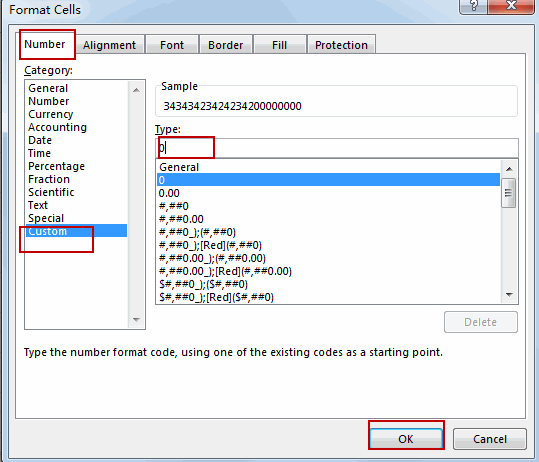#4 Let’s see the result: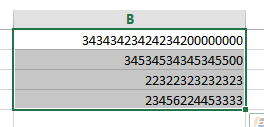You can also use an excel formula to convert scientific notation to text based on the TRIM function or the UPPER function.

Type the following formula into a blank cell and then press Enter key.

=TRIM(B1)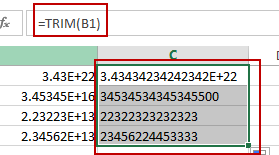Or

=UPPER(B1)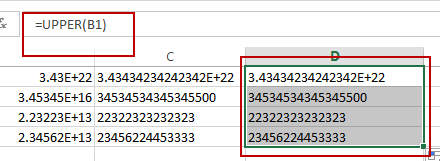There is a simple method to convert scientific notation to text, you just need to type a single quote before the scientific notation number. Then it will show the normal number.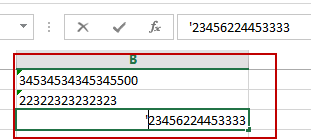### Related Functions

• Excel TRIM function
The Excel TRIM function removes all spaces from text string except for single spaces between words.  You can use the TRIM function to remove extra spaces between words in a string.The syntax of the TRIM function is as below:= TRIM (text)….
• Excel UPPER function
The Excel UPPER function converts all characters in text string to uppercase.The UPPER function is a build-in function in Microsoft Excel and it is categorized as a Text Function.The syntax of the UPPER function is as below:= UPPER (text)…

## Change Uppercase and Lowercase Text

This post will guide you how to change the case of text to uppercase or lowercase in Excel. How do I change all uppercase text to lower case with an Excel formula.

For example, if you want to change all text string in uppercase into lowercase, you can create a formula based on the Lower function. And if you want to convert all letters in one cell into uppercase, you can use the Upper function as a formula in excel.

If you want to show in Cell C1 a lowercase version of the text in Cell B1, you can type the following formula into the formula box in Cell C1.

=LOWER(B1)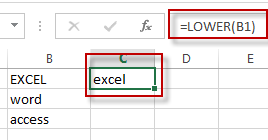If you want to show in Cell C2 an uppercase version of the text in B2, you could type the below formula into the formula box in C2.

=UPPER(B2)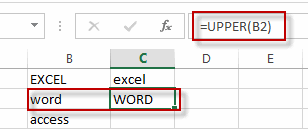If you want to show in Cell C3 a proper version of the text in B3, you could type the below formula into the formula box in C3:

=PROPER(B3)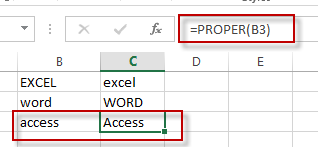### Related Functions

• Excel UPPER function
The Excel UPPER function converts all characters in text string to uppercase.The UPPER function is a build-in function in Microsoft Excel and it is categorized as a Text Function.The syntax of the UPPER function is as below:= UPPER (text)…
• Excel LOWER function
The Excel LOWER function converts all characters in a text string to lowercase.The syntax of the LOWER function is as below:= LOWER  (text)…
• Excel Proper Function
The Excel PROPER function capitalizes the first character in each word of a text string and set other characters to lowercase. The syntax of the PROPER function is as below:= PROPER  (text)…

## How to Check If the First Letter is capitalized

This post explains that how to check if the first letter in a cell is capitalized or not in excel. How to write an excel Macro to check if the first letter in a specified cell is capitalized or not. How to test if the first letter in a table cell is capitalized with VBA macro in excel. How to capitalize the first letter in a cell with Excel formula.

## Check if the first letter is or not capitalized

To check if the first letter in a text string is or not capitalized, you can write an Excel macro to achieve it. So you can follow the below steps to check the first letter:

1# click on “Visual Basic” command under DEVELOPER Tab.2# then the “Visual Basic Editor” window will appear.

3# click “Insert” ->”Module” to create a new module4# paste the below VBA code into the code window. Then clicking “Save” button.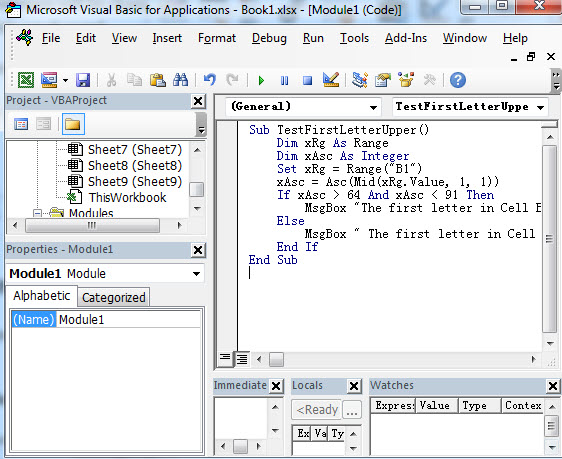```Sub TestFirstLetterUpper()
Dim xRg As Range
Dim xAsc As Integer
Set xRg = Range("B1")
xAsc = Asc(Mid(xRg.Value, 1, 1))
If xAsc > 64 And xAsc < 91 Then
MsgBox "The first letter in Cell B1 is capitalized."
Else
MsgBox " The first letter in Cell B1 is not capitalized "
End If
End Sub```

This VBA code will check if the first letter in Cell B1 is capitalized or not. So you just need to change it to other cells or range as you need.

Or you can use another VBA Macro to achieve the same result:

```Sub TestFirstLetterUpper2()
var1 = "B1"
If UCase(Left(var1, 1)) = Left(var1, 1) Then
MsgBox "First letter capitalized"
Else
MsgBox "First letter not capitalized"
End If
End Sub```

5# back to the current worksheet, then run the above excel macro.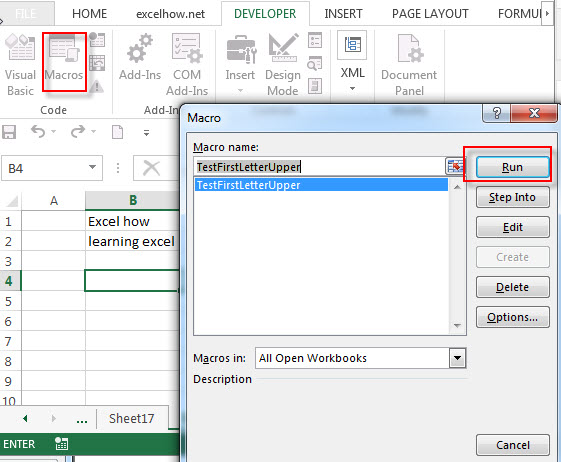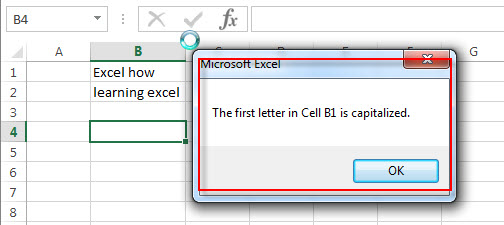## Capitalize the first letter in a Cell

Normally, it is very easy to capitalize all letters in a cell in excel, you just need to use the PROPER function to convert it.

`=PROPER(B1)`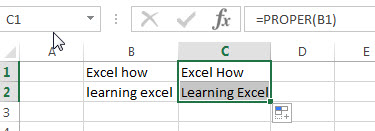If you want to capitalize only the first letter in a cell, you can create an excel formula based on the REPLACE function, the UPPER function and the LEFT function.

Assuming that you’d like to capitalize the first letter in a first word in Cell B1, then you can write down the following formula:

`=REPLACE(B1,1,1, UPPER(LEFT(B1,1)))`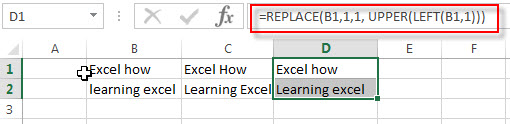You can also use another excel formula to achieve the same result as follows:

`=CONCATENATE(UPPER(LEFT(B1,1)), RIGHT(B1,LEN(B1)-1))`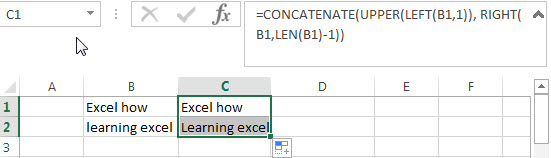### Related Functions

• Excel LEN function
The Excel LEN function returns the length of a text string (the number of characters in a text string).The LEN function is a build-in function in Microsoft Excel and it is categorized as a Text Function.The syntax of the LEN function is as below:= LEN(text)…
• Excel RIGHT function
The Excel RIGHT function returns a substring (a specified number of the characters) from a text string, starting from the rightmost character.The syntax of the RIGHT function is as below:= RIGHT (text,[num_chars])…
• Excel Replace function
The Excel DATE function returns the serial number for a date.The syntax of the DATE function is as below:= DATE (year, month, day) …
• Excel LEFT function
The Excel LEFT function returns a substring (a specified number of the characters) from a text string, starting from the leftmost character.The LEFT function is a build-in function in Microsoft Excel and it is categorized as a Text Function.The syntax of the LEFT function is as below:= LEFT(text,[num_chars])…t)…
• Excel UPPER function
The Excel UPPER function converts all characters in text string to uppercase.The UPPER function is a build-in function in Microsoft Excel and it is categorized as a Text Function.The syntax of the UPPER function is as below:= UPPER (text)…
• Excel Proper Function
The Excel PROPER function capitalizes the first character in each word of a text string and set other characters to lowercase. The syntax of the PROPER function is as below:= PROPER  (text)…
• Excel Concat function
The excel CONCAT function combines 2 or more strings or ranges together.This is a new function in Excel 2016 and it replaces the CONCATENATE function.The syntax of the CONCAT function is as below:=CONCAT (text1,[text2],…)…

## Excel Upper Function

This post will guide you how to use Excel UPPER function with syntax and examples in Microsoft excel.

### Description

The Excel UPPER function converts all characters in text string to uppercase.

The UPPER function is a build-in function in Microsoft Excel and it is categorized as a Text Function.

The UPPER function is available in Excel 2016, Excel 2013, Excel 2010, Excel 2007, Excel 2003, Excel XP, Excel 2000.

### Syntax

The syntax of the UPPER function is as below:

= UPPER (text)

Where the UPPER function arguments are:
Text -This is a required argument. The text that you want to converted to uppercase.

### Example

The below examples will show you how to use Excel UPPER Text function to convert all characters in text string to uppercase.

#1 To convert all characters to uppercase in B1 cell, just using formula:= UPPER(B1).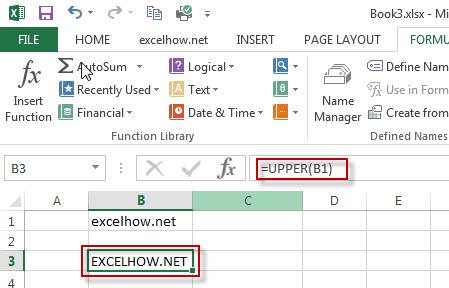### More Related UPPER Function Examples

• Change Uppercase and Lowercase Text
if you want to change all text string in uppercase into lowercase, you can create a formula based on the Lower function. And if you want to convert all letters in one cell into uppercase, you can use the Upper function as a formula in excel.…